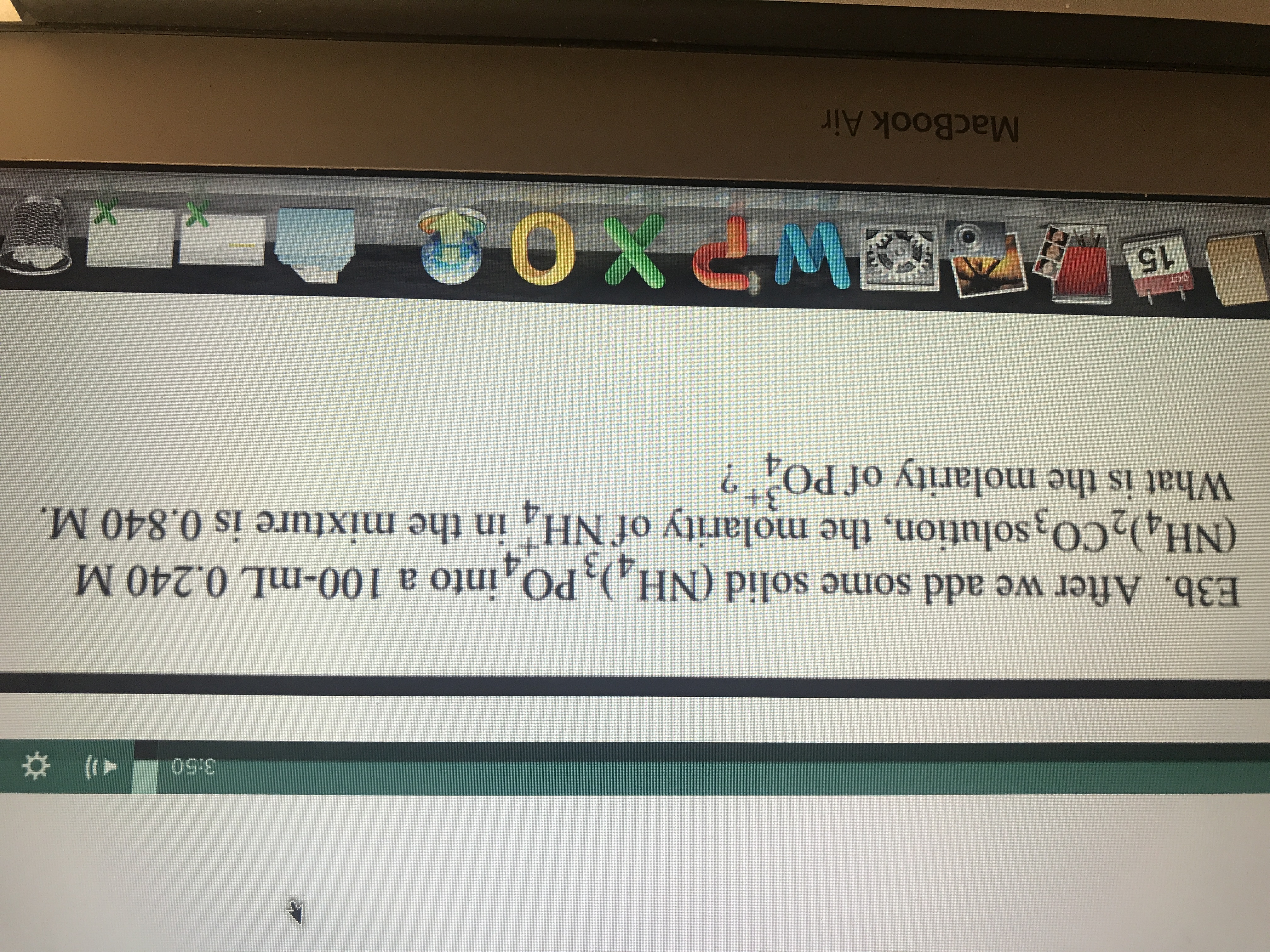# )3:50E3b. After we add some solid (NH,), PO, into a 100-mL 0.240 M(NH4)2CO3 solution, the molarity of NH in the mixture is 0.840 M.What is the molarity of PO43+ANDXOOCE15DOTMacBook Air

Question
69 viewshelp_outlineImage Transcriptionclose) 3:50 E3b. After we add some solid (NH,), PO, into a 100-mL 0.240 M (NH4)2CO3 solution, the molarity of NH in the mixture is 0.840 M. What is the molarity of PO 4 3+ ANDXOO CE 15 DOT MacBook Air fullscreen
check_circle

star
star
star
star
star
1 Rating
Step 1

The molarity of a solution is the ratio of number of moles of a substance to the volume of solution in liters. The expression for molarity is labelled in equation (1) in which M is the molarity, n is the number of moles of solute and  V is the volume in liters.

The relation between millilitres (mL) and liters (L) is written below:

1 mL= 0.001 L

Step 2

Convert 100 mL into liters by using equation (2).

100 mL = 0.100 L

The number of moles of ammonium carbonate is calculated below.

Step 3

The number of moles of the mixt...

### Want to see the full answer?

See Solution

#### Want to see this answer and more?

Solutions are written by subject experts who are available 24/7. Questions are typically answered within 1 hour.*

See Solution
*Response times may vary by subject and question.
Tagged in

### General Chemistry